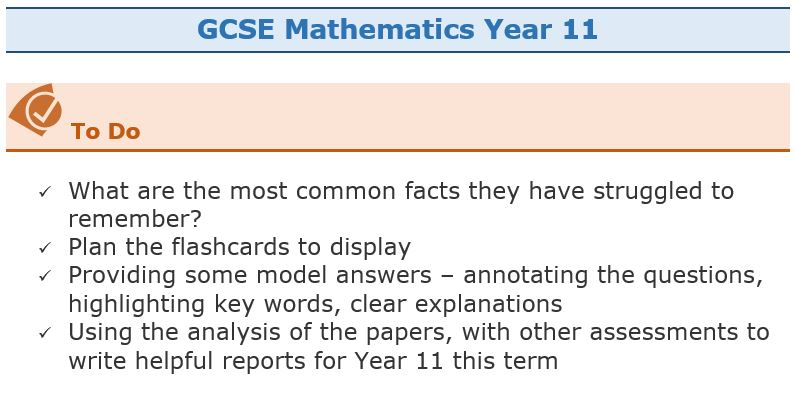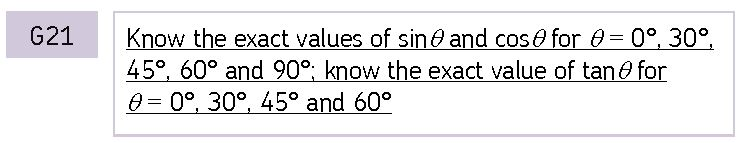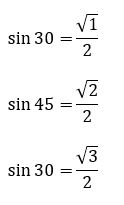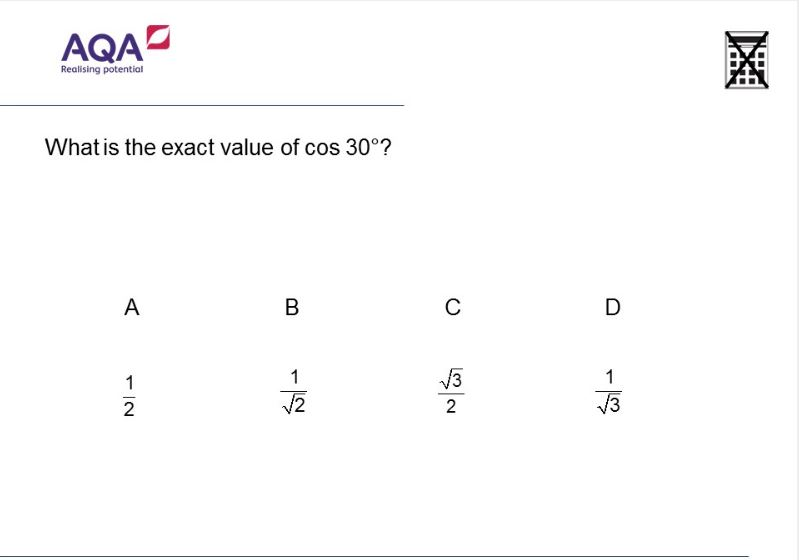# GCSE Mathematics

Marking GCSE Mock Examinations, I wrote myself a To Do List:Completing the question by question mark entry for each of the three papers as mentioned in the previous post has shown some clear trends as to facts students have recalled incorrectly. I plan to check that students can accurately recall facts, terminology and definitions (A01) as a regular part of lessons, even for a few minutes. Clearly to achieve highly on questions requiring students to reason, interpret and communicate mathematically (A02) and Solve problems within mathematics and in other contexts (A03) students do need easy recall of all these facts.

Resources to help with this aim include Flashcards on Tanner Maths, A4 or A5 size cards are available. For use in lessons I intend to project flashcards of my choice. Also useful here is Mathscard from Loughborough University; whilst written for A Level, several parts are useful for GCSE. The online version can easily be projected.

If we look at Trigonometry for example you will see that the graphs are included as are the standard triangles used to find exact values of angles.

I plan to ask the questions of students in the form of a mini test, so I will ask students the question and they just write down the answer, I can then display that answer. For trigonometry for example I will ask them to draw sketches of the standard triangles illustrated here. Appropriate flashcards can be displayed whilst asking the questions. An alternative would be the syllabus itself or a document such as AQA’s Teaching Guidance illustrated below. For example, from AQA we have:As Howard has pointed out in his comment below – note the structure of the values for sine and cosine!

Another possibility will be to use the Examination collections from Diagnostic Questions.These resources can of course all be made available to students so they can quiz themselves at home. This could in fact be a regular part of a homework.

Revision will be a regular feature in lessons. As my Year 11 students are studying AQA’s Level 2 Further Maths, the overlap between this and the GCSE specification will help them.

Revision Resources can be found here and another very varied selection here.

For the formulae that need to be known and understood – see these slides.

## 3 comments on “GCSE Mathematics”

1.howardat58

And, surprise, surprise, sin 90 is root(4)/2 = 1
(Just figured this one out)

•howardat58

Finally we have sin 0 is root(0)/2 which is 0

2.howardat58

Just to highlight the structure of the sines and cosines, check this:
sin 30 is 1/2
sin 45 is root(2)/2
sin 60 is root(3)/2

and better still:
sin 30 is root(1)/2
sin 45 is root(2)/2
sin 60 is root(3)/2

Can’t do square root signs here!